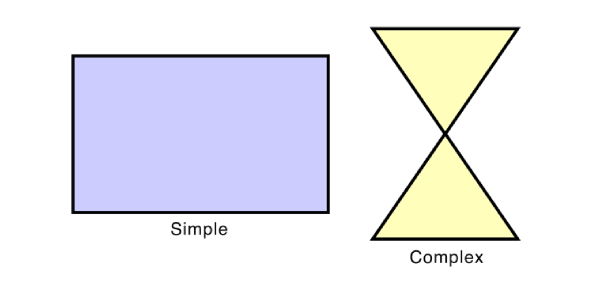# Math Quiz: Fractions, Decimals And Quadrilaterals

Approved & Edited by ProProfs Editorial Team
At ProProfs Quizzes, our dedicated in-house team of experts takes pride in their work. With a sharp eye for detail, they meticulously review each quiz. This ensures that every quiz, taken by over 100 million users, meets our standards of accuracy, clarity, and engagement.
| Written by Jmylesja
J
Jmylesja
Community Contributor
Quizzes Created: 1 | Total Attempts: 279
Questions: 10 | Attempts: 279SettingsAn assessment of topics covered in class: fractions, decimals and quadrilaterals.

• 1.

### For the number 25.639, what is the place value of the digit 6?

• A.

6 tens

• B.

6 tenths

• C.

6 units

• D.

6 hundredths

B. 6 tenths
Explanation
The place value of a digit determines its position in a number. In the number 25.639, the digit 6 is in the tenths place. This means that it represents a value of 6 tenths, or 0.6.

Rate this question:

• 2.

### What is the hundredths digit in the number 356.812?

• A.

1

• B.

2

• C.

3

• D.

8

A. 1
Explanation
The hundredths digit in the number 356.812 is 1. This is because the hundredths place is the second digit after the decimal point, and in this case, it is 1.

Rate this question:

• 3.

### Which of the following numbers has a 5 in the thousandths place?

• A.

5,003.256

• B.

576.835

• C.

89.0527

• D.

31.8605

B. 576.835
Explanation
The number 576.835 has a 5 in the thousandths place. In this number, the digit 5 is located after the decimal point and before the digit 8, which is in the hundredths place. The digits after the 5 are 3 and 5, which are in the thousandths and ten-thousandths places, respectively. Therefore, the number 576.835 satisfies the condition of having a 5 in the thousandths place.

Rate this question:

• 4.

### What is the name of this quadrilateral?

• A.

Kite

• B.

Square

• C.

Rhombus

• D.

Trapezoid or Trapezium

B. Square
Explanation
The name of the quadrilateral is a square because it has four equal sides and four right angles. A square is a special type of rectangle where all sides are equal in length.

Rate this question:

• 5.

### What is the name of this quadrilateral?

• A.

Trapezoid or Trapezium

• B.

Parallelogram

• C.

Kite

• D.

Rhombus

C. Kite
Explanation
A kite is a quadrilateral with two pairs of adjacent sides that are equal in length. The shape of a kite resembles that of a kite used in flying. It has two pairs of consecutive sides that are equal in length, but the opposite sides are not parallel. Therefore, the correct answer for the name of this quadrilateral is kite.

Rate this question:

• 6.

• A.

35°

• B.

45°

• C.

65°

• D.

55°

D. 55°
• 7.

### A                        B                         C                         D

• A.

3/4

• B.

1/4

• C.

4/1

• D.

2/4

B. 1/4
Explanation
The correct answer is 1/4. This can be determined by simplifying the fraction 3/4. When we divide the numerator (3) by the denominator (4), we get 0.75. Therefore, the fraction 3/4 is equivalent to the decimal 0.75. Since the answer is asking for a fraction, we convert the decimal 0.75 to a fraction by writing it as 75/100. We can simplify this fraction by dividing both the numerator and denominator by their greatest common divisor, which is 25. After simplifying, we get 3/4. Therefore, the fraction 3/4 is equal to 1/4.

Rate this question:

• 8.

### A                           B                       C                          D

• A.

6/5

• B.

1/6

• C.

3/5

• D.

5/6

D. 5/6
Explanation
The given answer is 5/6. This means that out of the four options provided (A, B, C, D), the fraction 5/6 is the correct answer.

Rate this question:

• 9.

2
• 10.

### Fill in the missing number to make an equivalent fraction.                   =      ______                  10Back to top